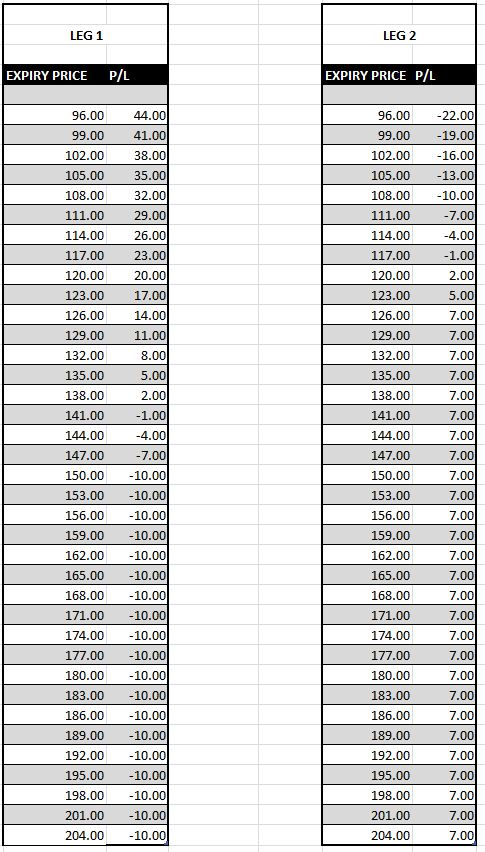Back

Categories

Learning

Option StrategiesA PUT spread is an options strategy in which equal number of Put option contracts are bought and sold simultaneously on the same underlying security but with different strike prices. Put spreads limit the option trader's maximum loss at the expense of capping his potential profit at the same time. In simple terms, you pay a cheaper Premium for less payout, if right. The max profit will be the difference between the two strike prices, less the premium paid. The downside for a Put Spread buyer remains the premium paid. This is what the profit and loss graph looks like;There are places that refer to these spreads as bull or bear spreads, this is because how call and put spreads can mirror each other. EG: buying a put spread is EXACTLY the same as selling a call spread (assuming you use the same strikes) So these are referred to as BULL SPREADS

Conversely, if you sell a put spread, it is EXACTLY the same as buying a call spread (assuming you use the same strikes) These are referred to as BEAR SPREADS

Throughout the learning section I will advise you to manually do an expiration Profit & Loss table. This allows you to visualize exactly what it is that you are doing and is something all beginner traders do to start learning about strategies.YOU then just need to work out each individual leg profit and loss then net it out in a graphWhy these terms? BULL is used when we think something is going UP and BEAR when we think it is going DOWN

## QUIZ TIME

Test your skills with our quiz and challenge the waves.

If I purchase a 125/150 put spread for \$7.50, what is the equivalent sell in the call spread to give me an identical P&L?

Option StrategiesDarren Krett

Thursday, 8 June 2023

A Call Spread is an options strategy in which equal number of Call option contracts are bought and sold simultaneously on the same underlyin

0